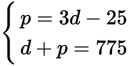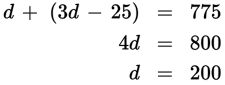# SAT Math Multiple Choice Question 563: Answer and Explanation

### Test Information

Question: 563

8. In the legal field, "reciprocity" means that an attorney can take and pass a bar exam in one state, and be allowed to practice law in a different state that permits such reciprocity. Each state bar association decides with which other states it will allow reciprocity. For example, Pennsylvania allows reciprocity with the District of Columbia. It costs \$25 less than 3 times as much to take the bar in Pennsylvania than in D.C. If both bar exams together cost \$775, how much less expensive is it to take the bar exam in D.C. than in Pennsylvania?

• A. \$200
• B. \$275
• C. \$375
• D. \$575

Explanation:

C

Difficulty: Medium

Category: Heart of Algebra / Systems of Linear Equations

Strategic Advice: Use the Kaplan Method for Translating English into Math. Write a system of equations with p = the cost in dollars of the Pennsylvania bar exam and d = the cost of the D.C. bar exam.

Getting to the Answer: The Pennsylvania bar exam (p) costs \$25 less (– 25) than 3 times as much (3d) as the D.C. bar exam, or p = 3d – 25. Together, both bar exams cost \$775, so d + p = 775. The system is:The top equation is already solved for p, so substitute 3d – 25 into the second equation for p, and solve for d:Be careful—that's not the answer. The D.C. bar exam costs \$200, which means the Pennsylvania bar exam costs \$775 – \$200 = \$575. This means the D.C. bar exam is \$575 – \$200 = \$375 less expensive than the Pennsylvania bar exam.